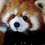# Primorial Numbers

We can define something similar to the factorial function, $$n!$$, for prime numbers. This is known as the "primorial" function and it written as $$p\#$$. It is defined by

$n \# = \displaystyle\prod_{p < n ,\: prime}^{n} p$

The first few values are:

$3\# = 2 \cdot 3 = 6$ $5\# = 30$ $7\# = 210$ $11\# = 2310$

They have an interesting property involving Euler's Totient function: $\frac{\phi (n \#)}{n \#} = \displaystyle\prod_{p < n ,\: prime}^{n} 1 - \frac{1}{p} \simeq \frac{1}{ln(n)}$

The second follows from the definition of the totient function while the third follows from the prime number theorem. What this means, essentially, is that the totient density of primorials is strictly decreasing to zero. Let me explain.

Primorials are important because they essentially "sieve" through prime numbers. For example, every prime number can also be written in the form of $6n \pm 1$. Likewise, they can be written in the forms $30n \pm 1, 30n \pm 7, 30n \pm 11, 30n \pm 13$. This continues for all other primorials.

But what's so special about those? We can also write primes as $4n \pm 1, 10n \pm (1, 3)$, and so on. What's really important is minimizing the number of residue classes we have. In this light, we can imagine that there are some numbers which work better than others. For example, the prime number residue classes of $7$ are $\pm 1, \pm 2, \pm 3$. So we could write prime numbers in the form of $7n \pm(1,2,3)$, but why bother when we can write them as $6n \pm 1$ and reduce the total number of residue classes from $\phi(7)=6$ to $\phi(6)=2$?

This is where the $\phi (n) / n$ term comes from in the equation above. It measures the number of residue classes of $n$ relative to its size. Primorials are important because they optimize this measure and occupy its minimum values.

A unique application of primorials is in calculating heuristics about the gaps between prime numbers. I'll develop this fully in a later note, but the general argument is as follows:

Take a prime number of the form $6n + x$ and write out the next prime that comes after it. There are 4 possibilities:

$\begin{array}{lr} prime \: 1 & prime \: 2 & gap \\ 6n+1 & 6k + 1 & 6s \\ 6n+1 & 6k + 5 & 6s + 4 \\ 6n+5 & 6k + 1 & 6s+2 \\ 6n+5 & 6k + 5 & 6s \end{array}$

The gap is divisible by $2$ in all cases, which is a trivial fact. In half of the cases, it is divisible by $3$. If we look at larger residue classes, we find that gaps are divisible by $5$ in a third of cases and so on. Assuming that s is minimal (looking only at twin primes, cousin primes, and sexy primes), we can also use this heuristic to state that sexy primes (prime pairs with a gap of $6$) are about twice as common as twin and cousin primes (those with gaps $2$ and $4$, respectively).Note by Levi Walker
1 year, 7 months ago

This discussion board is a place to discuss our Daily Challenges and the math and science related to those challenges. Explanations are more than just a solution — they should explain the steps and thinking strategies that you used to obtain the solution. Comments should further the discussion of math and science.

When posting on Brilliant:

• Use the emojis to react to an explanation, whether you're congratulating a job well done , or just really confused .
• Ask specific questions about the challenge or the steps in somebody's explanation. Well-posed questions can add a lot to the discussion, but posting "I don't understand!" doesn't help anyone.
• Try to contribute something new to the discussion, whether it is an extension, generalization or other idea related to the challenge.

MarkdownAppears as
*italics* or _italics_ italics
**bold** or __bold__ bold
- bulleted- list
• bulleted
• list
1. numbered2. list
1. numbered
2. list
Note: you must add a full line of space before and after lists for them to show up correctly
paragraph 1paragraph 2

paragraph 1

paragraph 2

[example link](https://brilliant.org)example link
> This is a quote
This is a quote
    # I indented these lines
# 4 spaces, and now they show
# up as a code block.

print "hello world"
# I indented these lines
# 4 spaces, and now they show
# up as a code block.

print "hello world"
MathAppears as
Remember to wrap math in $$ ... $$ or $ ... $ to ensure proper formatting.
2 \times 3 $2 \times 3$
2^{34} $2^{34}$
a_{i-1} $a_{i-1}$
\frac{2}{3} $\frac{2}{3}$
\sqrt{2} $\sqrt{2}$
\sum_{i=1}^3 $\sum_{i=1}^3$
\sin \theta $\sin \theta$
\boxed{123} $\boxed{123}$

Sort by:

So, if the heuristic relates the occurences of prime pairs with gaps 2, 4, 6, ..., would a solution to the twin prime conjecture also prove that there are infinitely many prime pairs with any gap? Or is the heuristic too inaccurate for that?

- 1 year, 7 months ago

It would strongly suggest it, but it's still too weak to prove it. For example, we currently know that there is at least 1 prime gap below 246 which occurs infinitely often, but not which one it is. If de Polignac's conjecture is true (I believe it is), then the existence of one infinitely frequent prime gap ensures the existence of all of them - but it's based solely on heuristic arguments so far and has yet to be proven rigorously.

- 1 year, 7 months ago

Ah, interesting. Your discussions always cover really nice bits of mathematics, and I admire you for your great ideas and how you present them!

- 1 year, 7 months ago

Aww, thanks! I try my best :)

- 1 year, 7 months ago